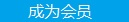您可以捐助，支持我们的公益事业。 1元 10元 50元 认证码：必填求知 文章 文库 Lib 视频 Code iProcess 课程 角色 咨询 工具 火云堂 讲座吧 建模者要资料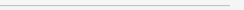Tensorflow卷积神经网络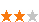1780 次浏览     评价： 好 中 差
2018-1-24

 编辑推荐: 本文来源Finley博客，本文将简单介绍其原理并分析Tensorflow官方提供的示例。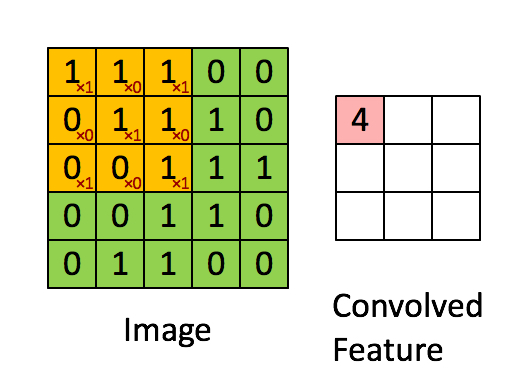1*1 + 1*0 + 1*1 + 0*0 + 1*1 + 1*0 + 0*1 + 0*0 + 1*1 = 4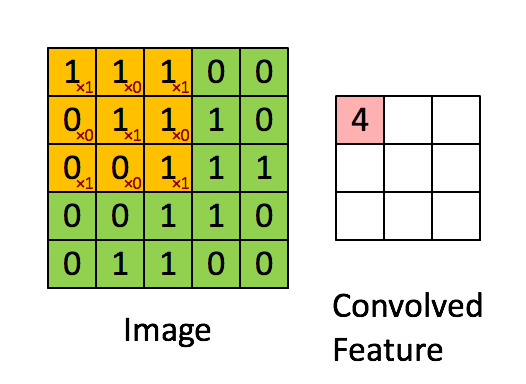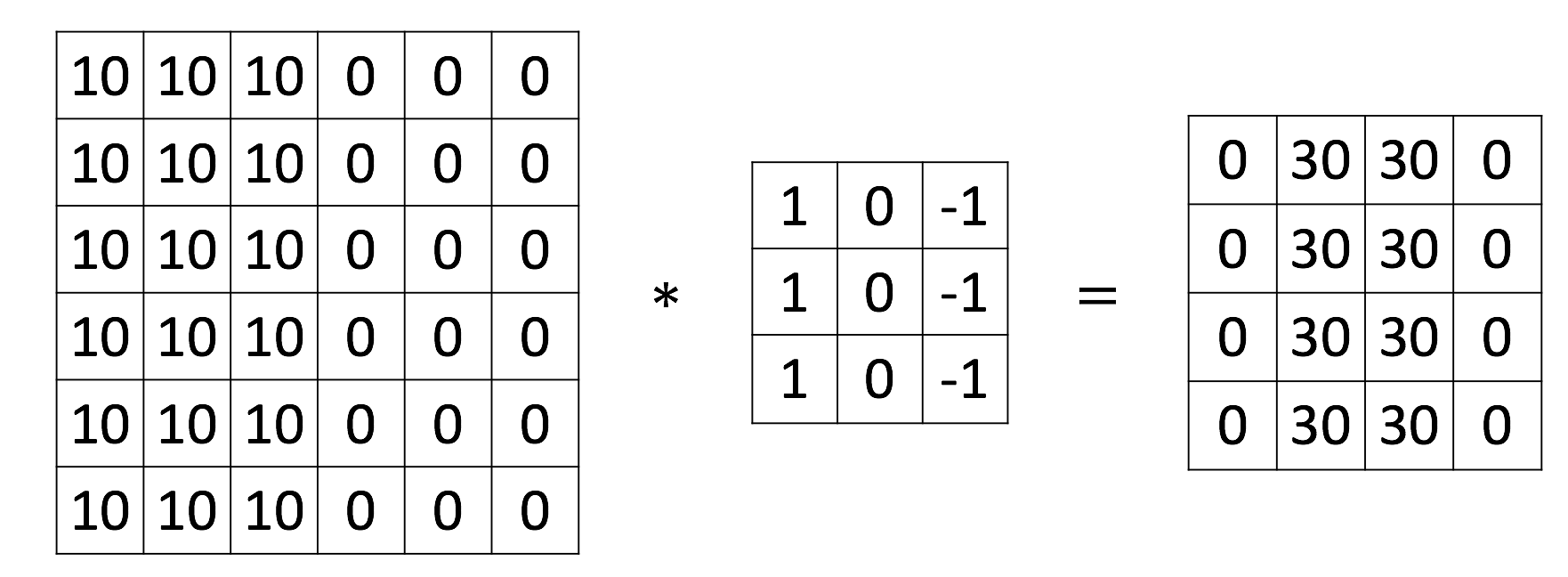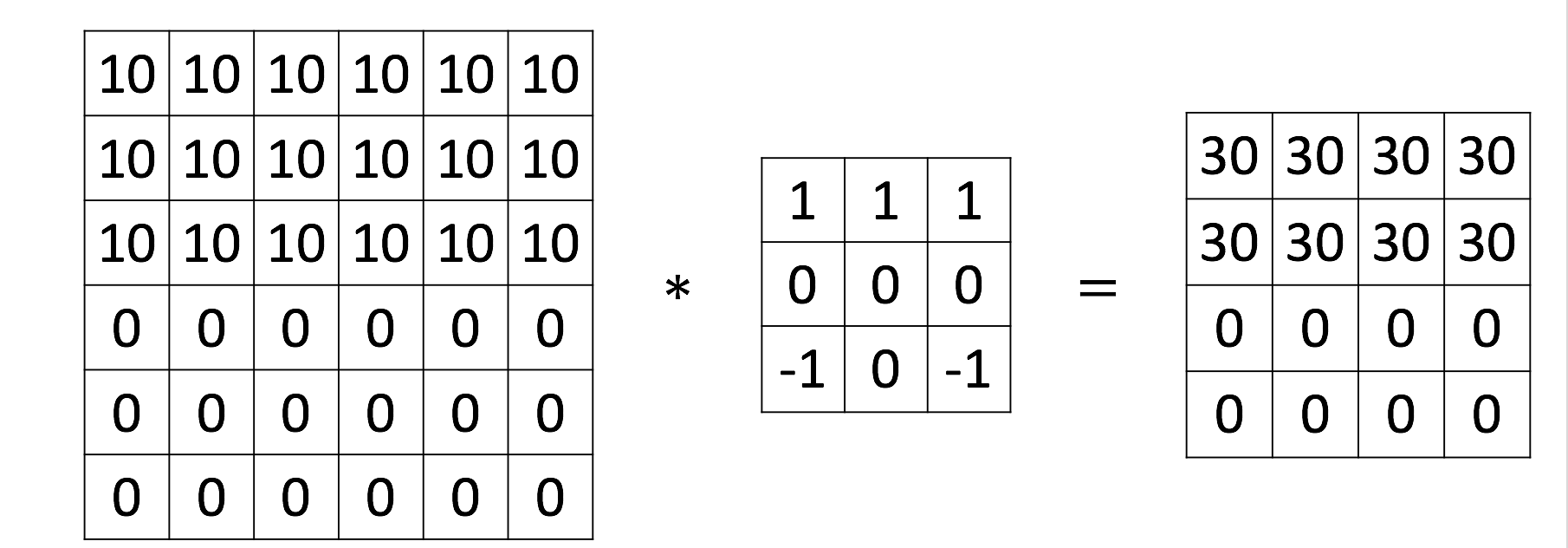• SAME: 使用附近点的值代替缺失的点, 可以保证特征图不会变小
• VALID: 只对可用的位置进行卷积(不进行填充), 但特征图会变小

SAME填充效果, 4*4矩阵被填充为6*6矩阵, 填充宽度p=1: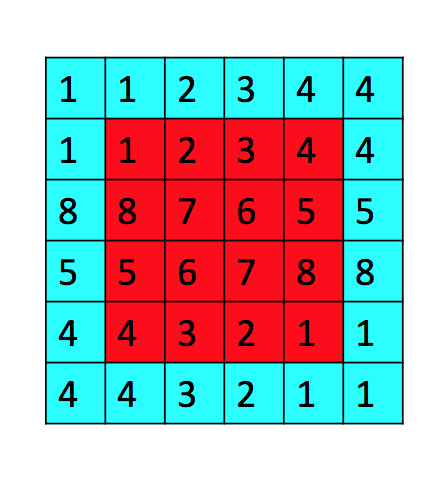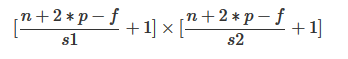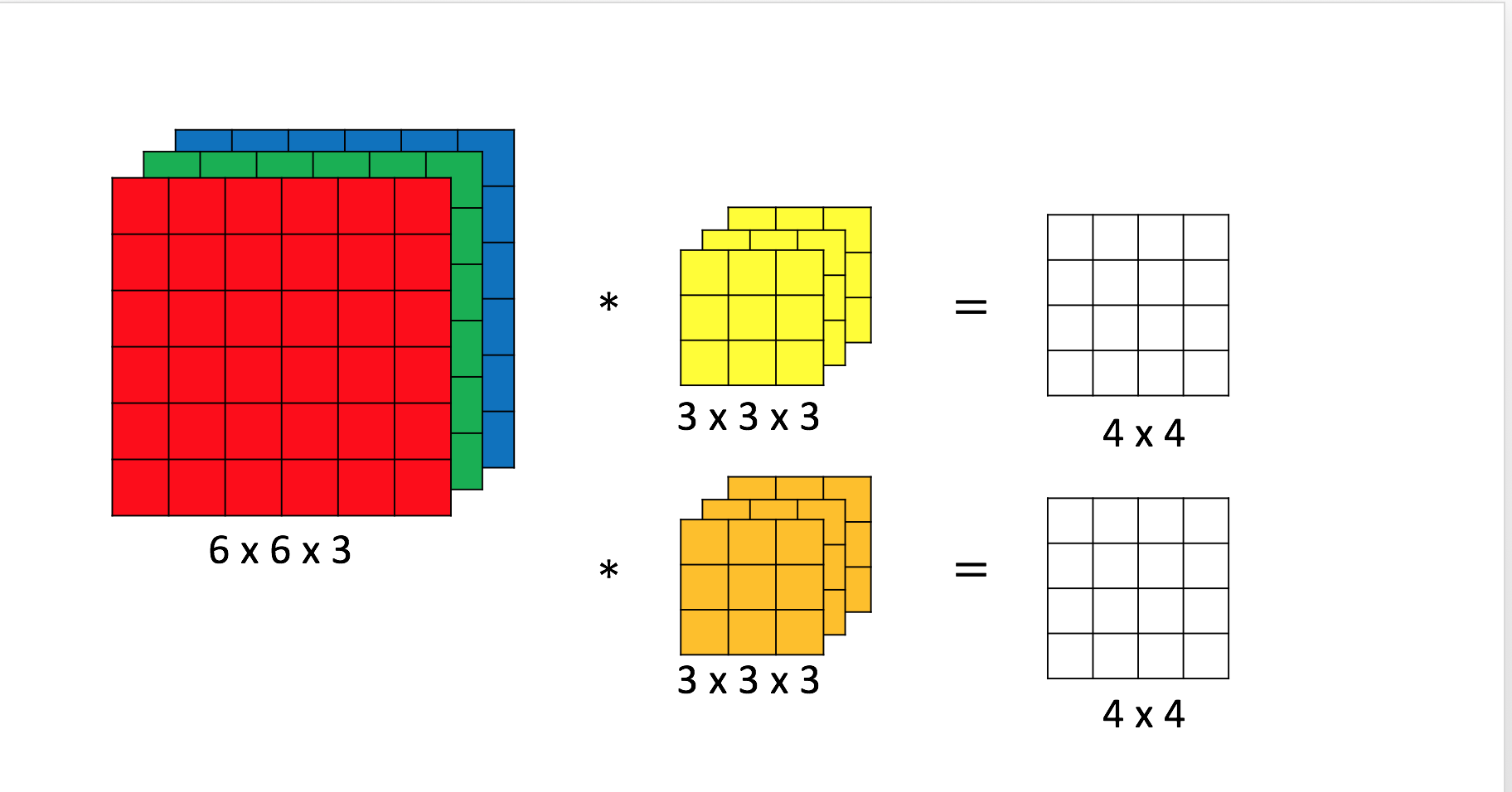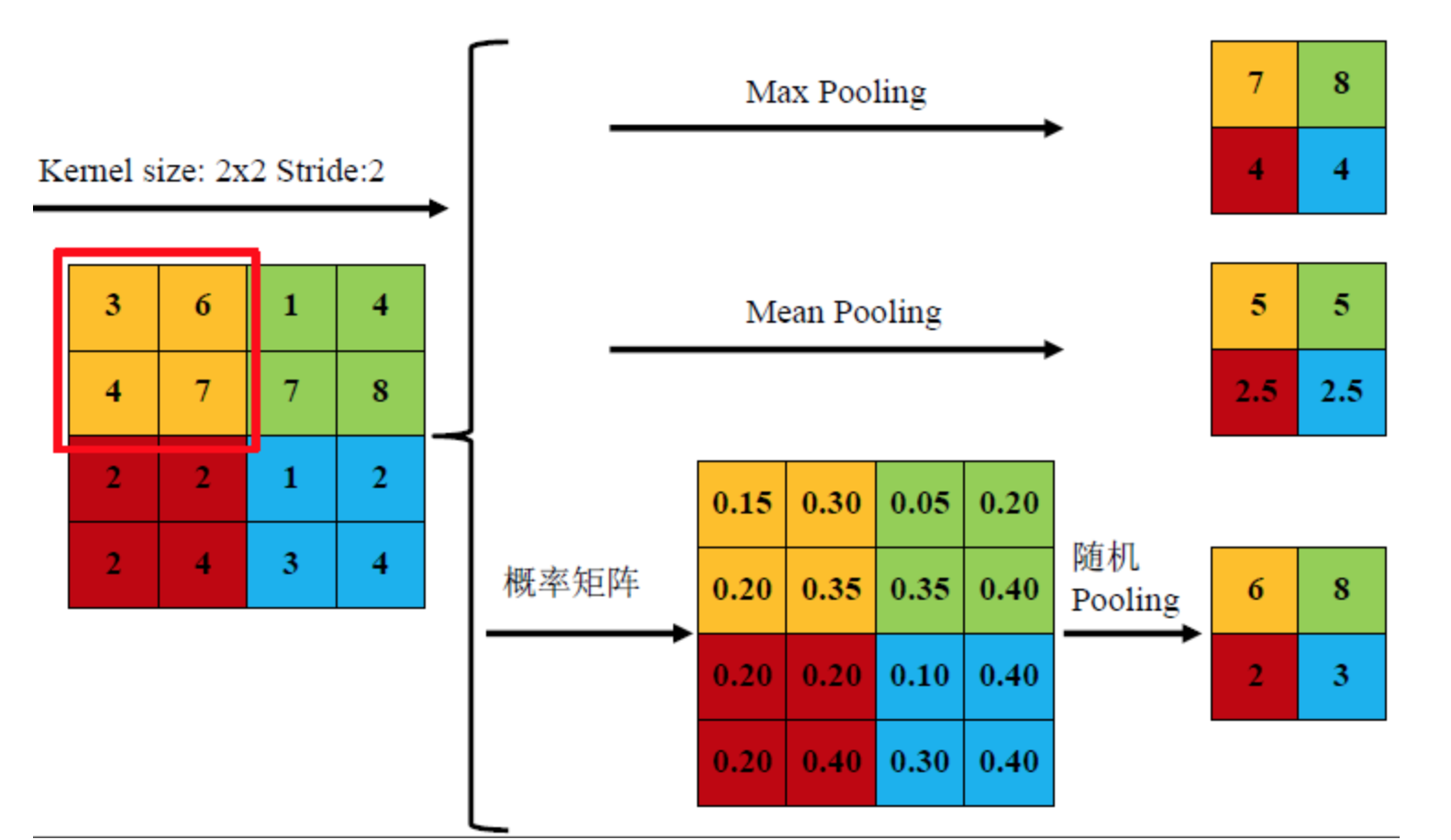TensorFlow实现

TensorFlow的文档Deep MNIST for Experts介绍了使用CNN在MNIST数据集上识别手写数字的方法., 该示例采用了LeNet5模型.

 import tempfile from tensorflow.examples.tutorials.mnist import input_data import tensorflow as tf

main(_)函数负责网络的构建:

 def main(_): # 导入MNIST数据集 # FLAGS.data_dir是本地数据的路径, 可以用空字符串代替以自动下载数据集 mnist = input_data.read_data_sets(FLAGS.data_dir, one_hot=True) # x是输入层, 每个28x28的图像被展开为784阶向量 x = tf.placeholder(tf.float32, [None, 784]) # y_是训练集预标注好的结果, 采用one-hot的方法表示10种分类 y_ = tf.placeholder(tf.float32, [None, 10]) # deepnn方法构建了一个cnn, y_conv是cnn的预测输出 # keep_prob是dropout层的参数, 下文再讲 y_conv, keep_prob = deepnn(x) # 计算预测y_conv和标签y_的交叉熵作为损失函数 with tf.name_scope('loss'): cross_entropy = tf.nn.softmax_cross_entropy_with_logits(labels=y_, logits=y_conv) cross_entropy = tf.reduce_mean(cross_entropy) # 使用Adam优化算法, 以最小化损失函数为目标 with tf.name_scope('adam_optimizer'): train_step = tf.train.AdamOptimizer(1e-4).minimize(cross_entropy) # 计算精确度(正确分类的样本数占测试样本数的比例), 用于评估模型效果 with tf.name_scope('accuracy'): correct_prediction = tf.equal(tf.argmax(y_conv, 1), tf.argmax(y_, 1)) correct_prediction = tf.cast(correct_prediction, tf.float32) accuracy = tf.reduce_mean(correct_prediction) main函数与其它tensorflow神经网络并无二致, 关键分析deepnn方法如何构建cnn: def deepnn(x): # x的结构为[n, 784], 将其展开为[n, 28, 28] # 灰度图只有一个通道, x_image第四维为1 # x_image的四维分别是[n_sample, width, height, channel] with tf.name_scope('reshape'): x_image = tf.reshape(x, [-1, 28, 28, 1]) # 第一个卷积层, 将28x28*1灰度图使用5*5*32核进行卷积 with tf.name_scope('conv1'): # 初始化连接权值, 为了避免梯度消失权值使用正则分布进行初始化 W_conv1 = weight_variable([5, 5, 1, 32]) # 初始化偏置值, 这里使用的是0.1 b_conv1 = bias_variable() # strides是卷积核移动的步幅. 采用SAME策略填充, 即使用相同值填充 # def conv2d(x, W): # tf.nn.conv2d(x, W, strides=[1, 1, 1, 1], padding='SAME') # h_conv1的结构为[n, 28, 28, 32] h_conv1 = tf.nn.relu(conv2d(x_image, W_conv1) + b_conv1) # 第一个池化层, 2*2最大值池化, 得到14*14矩阵 with tf.name_scope('pool1'): h_pool1 = max_pool_2x2(h_conv1) # 第二个卷积层, 将28*28*32特征图使用5*5*64核进行卷积 with tf.name_scope('conv2'): W_conv2 = weight_variable([5, 5, 32, 64]) b_conv2 = bias_variable() # h_conv2的结构为[n, 14, 14, 64] h_conv2 = tf.nn.relu(conv2d(h_pool1, W_conv2) + b_conv2) # 第二个池化层, 2*2最大值池化, 得到7*7矩阵 with tf.name_scope('pool2'): # h_pool2的结构为[n, 7, 7, 64] h_pool2 = max_pool_2x2(h_conv2) # 第一个全连接层, 将7*7*64特征矩阵用全连接层映射到1024个特征 with tf.name_scope('fc1'): W_fc1 = weight_variable([7 * 7 * 64, 1024]) b_fc1 = bias_variable() h_pool2_flat = tf.reshape(h_pool2, [-1, 7*7*64]) h_fc1 = tf.nn.relu(tf.matmul(h_pool2_flat, W_fc1) + b_fc1) # 使用dropout层避免过拟合 # 即在训练过程中的一次迭代中, 随机选择一定比例的神经元不参与此次迭代 # 参与迭代的概率值由keep_prob指定, keep_prob=1.0为使用整个网络 with tf.name_scope('dropout'): keep_prob = tf.placeholder(tf.float32) h_fc1_drop = tf.nn.dropout(h_fc1, keep_prob) # 第二个全连接层, 将1024个特征映射到10个特征, 即10个分类的one-hot编码 # one-hot编码是指用 `100`代替1, `010`代替2, `001`代替3... 的编码方式 with tf.name_scope('fc2'): W_fc2 = weight_variable([1024, 10]) b_fc2 = bias_variable() y_conv = tf.matmul(h_fc1_drop, W_fc2) + b_fc2 return y_conv, keep_prob

main函数与其它tensorflow神经网络并无二致, 关键分析deepnn方法如何构建cnn:

 graph_location = tempfile.mkdtemp() print('Saving graph to: %s' % graph_location) train_writer = tf.summary.FileWriter(graph_location) train_writer.add_graph(tf.get_default_graph())

• 输入层x[n, 784]
• 输出层y_conv[n, 10]
• dropout保留比例keep_prob

 with tf.Session() as sess: # 初始化全局变量 sess.run(tf.global_variables_initializer()) for i in range(10000): # 每次取训练数据集中50个样本, 分10000次取出 # batch为特征集, 结构为[50, 784]即50组784阶向量 # batch为标签集, 结构为[50, 10]即50个采用one-hot编码的标签 batch = mnist.train.next_batch(50) # 每进行100次迭代评估一次精度 if i % 100 == 0: train_accuracy = accuracy.eval(feed_dict={ x: batch, y_: batch, keep_prob: 1.0}) print('step %d, training accuracy %g' % (i, train_accuracy)) # 进行训练, dropout keep prob设为0.5 train_step.run(feed_dict={x: batch, y_: batch, keep_prob: 0.5}) # 评估最终精度, dropout keep prob设为1.0即使用全部网络 print('test accuracy %g' % accuracy.eval(feed_dict={ x: mnist.test.images, y_: mnist.test.labels, keep_prob: 1.0}))

 if __name__ == '__main__': parser = argparse.ArgumentParser() parser.add_argument('--data_dir', type=str, default='/tmp/tensorflow/mnist/input_data', help='Directory for storing input data') FLAGS, unparsed = parser.parse_known_args() tf.app.run(main=main, argv=[sys.argv] + unparsed)

Keep working, we will find a way out. This is Finley, welcome to join us.1780 次浏览  评价: 好 中 差订阅捐助
 相关文章 我们该如何设计数据库 数据库设计经验谈 数据库设计过程 数据库编程总结
 相关文档 数据库性能调优技巧 数据库性能调整 数据库性能优化讲座 数据库系统性能调优系列
 相关课程 高性能数据库设计与优化 高级数据库架构师 数据仓库和数据挖掘技术 Hadoop原理、部署与性能调优每天2个文档/视频 扫描微信二维码订阅
 订阅技术月刊 获得每月300个技术资源